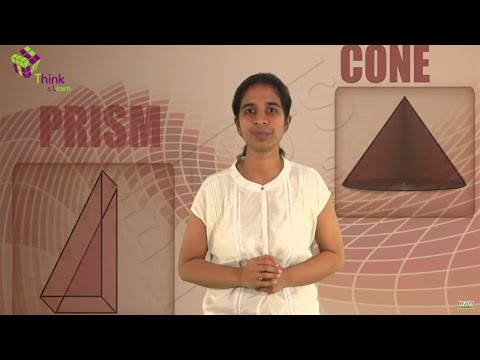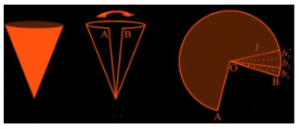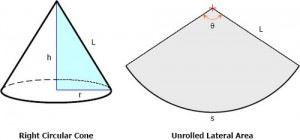# Surface Area Of A ConeCone is defined as a three dimensional solid structure that has a circular base. A cone can be viewed as a set of non-congruent circular disks that are placed over one another such that the ratio of radius of adjacent disks remain constant. Consider a cone as a triangle which is being rotated about one of its vertex. Now, think of a scenario where we need to paint the faces of a conical flask. Before painting, we need to know the quantity that is required to paint all the walls. We need to know the area every face of the container to determine the quantity of paint required and this is known as total surface area. Total surface area of a cone is the sum of areas of its faces. Let’s derive a general Cone formula to calculate the surface area of a cone.

Take a paper cone and cut it along its slant height to observe the figure being formed by the surface of the cone. Mark the two end points as A and B and the point of the intersection of lines as O.surface area of a cone

If you further cut this figure into multiple pieces viz. Ob1, Ob2, Ob3, …….., Obn each measuring the same length as the slant height of original cone, you will observe n triangles are formed out of it. Now, if you try calculating the total area of this figure, you just need to add area of these individual triangles. Hence,

Area of figure = (1/2) × (b1 + b2 + b3 + ………….. + bn)

=  (1/2)× (length of entire curved boundary)

Length of entire curved boundary = circumference of base = 2?× r (where r is the radius of the base)

Thus, area of figure =  $\frac{1}{2} \times 2 \pi \times r \times l= \pi rl$

Hence, curved surface area of a cone = $\pi rl$

Total Surface Area of a Cone-

The Total surface area of a cone includes the curved surface as well as area of its base, which is given as-

Base Area = $\pi r^{2}$

Curved Surface Area = $\pi rl$

Thus total surface area is given by-

$\pi r^{2} + \pi r l = \pi r (r+ l)$

Right Circular ConeA circular cone is the one with circular right section. A Right circular cone is the circular cone whose axis is perpendicular to the base.

## Examples

1. Determine the curved surface area of a cone whose base radius is 7 cm and a slant height is 15 cm.

Solution: Curved surface area of a cone = ?rl

= (22/7)× 7 ×15

=  330 cm2

2. Calculate the Total surface area of a cone whose radius is 8 cm and height is 12 cm.

Solution- We know that the total surface area is given as

$\pi r (r+ l)$

Also, $l = \sqrt{h^{2}+ r^{2}}$

Also, $l = \sqrt{8^{2}+ 12^{2}} = \sqrt{225} = 15$

Thus Total Surface Area $= \pi (8) (8 + 15)$

$= \pi (8) (23)$

$= 184 \pi$